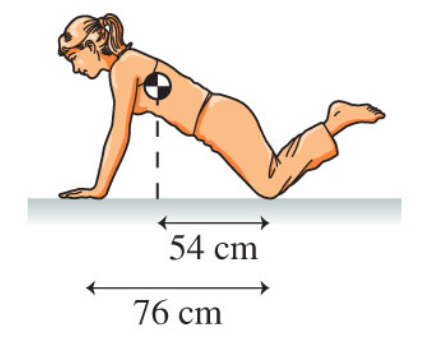# Problem: A woman weighing 670 N does a pushup from her knees, as shown in the figure. (Figure 1) Part A What are the normal forces of the floor on each of her hands? Express your answer to two significant figures and include the appropriate units. Part B What are the normal forces of the floor on each of her knees? Express your answer to two significant figures and include the appropriate units.

###### FREE Expert Solution

Under equilibrium, net torque is zero:

$\overline{){\mathbf{\Sigma }}{\mathbf{\tau }}{\mathbf{=}}{\mathbf{0}}}$

Similarly, the net force is zero:

$\overline{){\mathbf{\Sigma F}}{\mathbf{=}}{\mathbf{0}}}$

Part A

The net torque about the legs of the woman is zero.

Therefore:

$\begin{array}{lcl}\mathbf{\Sigma }\mathbf{\tau }& \mathbf{=}& \mathbf{w}\mathbf{\left(}\mathbf{54}\mathbf{×}{\mathbf{10}}^{\mathbf{-}\mathbf{2}}\mathbf{\right)}\mathbf{-}{\mathbf{F}}_{\mathbf{N}}\mathbf{\left(}\mathbf{76}\mathbf{×}{\mathbf{10}}^{\mathbf{-}\mathbf{2}}\mathbf{\right)}\mathbf{=}\mathbf{0}\\ {\mathbf{F}}_{\mathbf{N}}\mathbf{\left(}\mathbf{76}\mathbf{×}{\mathbf{10}}^{\mathbf{-}\mathbf{2}}\mathbf{\right)}& \mathbf{=}& \mathbf{w}\mathbf{\left(}\mathbf{54}\mathbf{×}{\mathbf{10}}^{\mathbf{-}\mathbf{2}}\mathbf{\right)}\\ {\mathbf{F}}_{\mathbf{N}}& \mathbf{=}& \frac{\mathbf{\left(}\mathbf{670}\mathbf{\right)}\mathbf{\left(}\mathbf{54}\mathbf{×}{\mathbf{10}}^{\mathbf{-}\mathbf{2}}\mathbf{\right)}}{\mathbf{\left(}\mathbf{76}\mathbf{×}{\mathbf{10}}^{\mathbf{-}\mathbf{2}}\mathbf{\right)}}\end{array}$

89% (47 ratings)###### Problem Details

A woman weighing 670 N does a pushup from her knees, as shown in the figure. (Figure 1)Part A What are the normal forces of the floor on each of her hands? Express your answer to two significant figures and include the appropriate units.

Part B What are the normal forces of the floor on each of her knees? Express your answer to two significant figures and include the appropriate units.